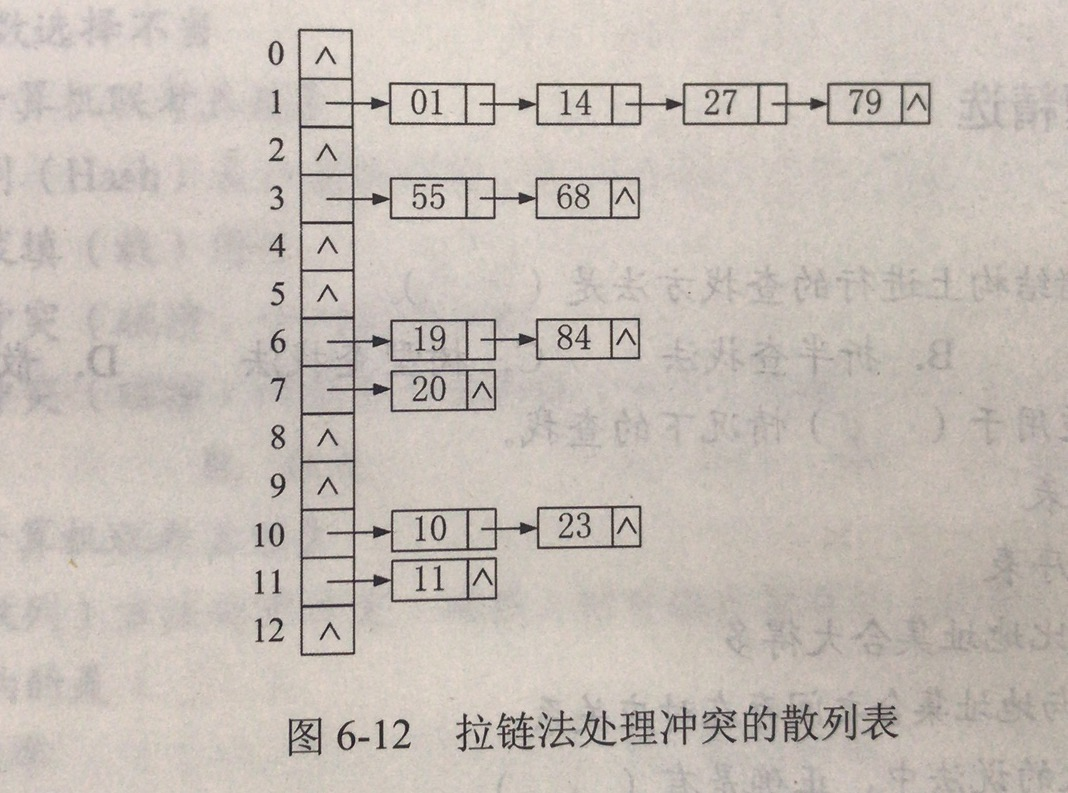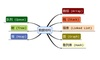• Console.WriteLine("********************哈希查找(C#版)********************\n"); //创建哈希表 for (int i = 0; i < list.Count; i++) { Insert(hashTable,list[i]); } Console.WriteLine("展示哈希...shell
• 哈希算法实现的C语言源程序 数据结构课程设计用
• 1、Hashing ...为了进一步降低算法的复杂度，构造一个新的数据结构， 能使得查找算法的复杂度降到O(1)， 这种概念称为“哈希Hashing” 能够使得查找的次数降低到常数级别， 我们对数据项所处的位置就必
1、Hashing
在文章《【数据结构与算法python】顺序查找算法的python实现（无序表）》与《【数据结构与算法python】顺序查找算法的python实现（有序表）中，我们利用数据集中关于数据项之间排列关系的知识， 来将查找算法进行了提升，如果数据项之间是按照大小排好序的话，就可以利用二分查找来降低算法复杂度。
为了进一步降低算法的复杂度，构造一个新的数据结构， 能使得查找算法的复杂度降到O(1)， 这种概念称为“哈希Hashing”
能够使得查找的次数降低到常数级别， 我们对数据项所处的位置就必须有更多的先验知识。 如果我们事先能知道要找的数据项应该出现在数据集中的什么位置， 就可以直接到那个位置看看数据项是否存在即可。
哈希表（hash table， 又称散列表） 是一种数据集， 其中数据项的存储方式尤其有利于将来快速的查找定位。
哈希表中的每一个存储位置， 称为槽（slot） ， 可以用来保存数据项， 每个槽有一个唯一的名称。
例如：一个包含11个槽的哈希表， 槽的名称分别为0～ 10
在插入数据项之前， 每个槽的值都是None， 表示空槽2、 哈希函数设计
（1）概念
我们引入“ 哈希函数”，用以根据数据项的值来确定其存放位置， 实现从数据项到存储槽名称的转换的， 称为 哈希函数（hash function）。
（2）设计原则
哈希函数不能成为存储过程和查找过程的计算负担，如果哈希函数设计太过复杂， 去花费大量的计算资源计算槽号，可能还不如简单地进行顺序查找或者二分查找，就失去了哈希本身的意义
（3）常用方法
①取余法
有一种常用的散列方法是“求余数”， 将数据项除以散列表的大小， 得到的余数作为槽号。实际上“求余数”方法会以不同形式出现在所有散列函数里，因为散列函数返回的槽号必须在散列表大小范围之内，所以一般会对散列表大小求余。
例子： 数据项： 54， 26， 93， 17， 77， 31；槽大小：11
本例中我们的散列函数是最简单的求余：h(item)= item % 11
按照散列函数h(item)，为每个数据项计算出存放的位置之后， 就可以将数
据项存入相应的槽中 ，对应关系如下所示②折叠法
折叠法设计散列函数的基本步骤是将数据项按照位数分为若干段，
再将几段数字相加，最后对散列表大小求余，得到散列值
例如， 对电话号码62767255，可以两位两位分为4段（62、 76、 72、 55），相加（62+76+72+55=265），散列表包括11个槽，那么就是265%11=1，所以h(62767255)=1
③折叠法（隔数反转 ）
有时候折叠法还会包括一个隔数反转的步骤，比如（62、 76、 72、 55）隔数反转为（62、 67、 72、 55），再累加（62+67+72+55=256），对11求余（256%11=3），所以h’(62767255)=3
虽然隔数反转从理论上看来毫无必要， 但这个步骤确实为折叠法得到散列函数提供了一种微调手段， 以便更好符合散列特性。
④平方取中法
平方取中法， 首先将数据项做平方运算，然后取平方数的中间两位， 再对散列表的大小求余，例如， 对44进行散列，首先44*44=1936，然后取中间的93，对散列表大小11求余， 93%11=5
⑤非数项
我们也可以对非数字的数据项进行散列，把字符串中的每个字符看作ASCII码即可，如cat， ord(‘c’)==99, ord(‘a’)==97,ord(‘t’)==116，再将这些整数累加（99+96+116=212），然后 对散列表大小求余（312%11=4）
当然， 这样的散列函数对所有的变位词（如car和rac，都将返回相同的散列值，为了防止这一点，可以将字符串所在的位置作为权重因子，乘以ord值再相加（（991+962+116*3=641）， 然后对散列表大小求余（641%11=3）
（4）负载因子
槽被数据项占据的比例称为散列表的“负载因子”
例子中的6个数据项插入后， 占据了散列表11个槽中的6个。
这里负载因子为6/11
（5）完美哈希函数
①概念
给定一组数据项， 如果一个哈希函数能把每个数据项映射到不同的槽中， 那么这个哈希函数就可以称为“完美哈希函数”，对于固定的一组数据，总是能想办法设计出完美哈希函数，但如果数据项经常性的变动， 很难有一个
系统性的方法来设计对应的完美散列函数。当然，冲突也不是致命性的错误，我们会有办法处理的。
②设计
获得完美哈希函数的一种方法是扩大哈希表的容量， 大到所有可能出现的数据项都能够占据不同的槽。 但这种方法对于可能数据项范围过大的情
况并不实用假如我们要保存手机号（11位数字），完美哈希函数得要求哈希表具有百亿个槽，会浪费太多存储空间。
好的哈希函数需要具备特性

冲突最少（近似完美）
计算难度低（额外开销小）
充分分散数据项（节约空间）

③用途（数据一致性校验 )
最著名的近似完美哈希函数是MD5和SHA系列函数。
MD5（Message Digest） 将任何长度的数据变换为固定长为128位（16字节）的“摘要” 。
SHA（Secure Hash Algorithm） 是另一组哈希函数。

SHA-0/SHA-1输出哈希值160位（20字节）
SHA-256/SHA-224分别输出256位、 224位
SHA-512/SHA-384分别输出512位和384位

为每个文件计算其哈希值， 仅对比其哈希值即可得知是否文件内容相同，有以下几种用途

网络文件下载完整性校验
文件分享系统：网盘中相同的文件（尤其是电影） 可以无需存储多次
防文件篡改：原理同数据文件一致性判断
彩票投注应用：彩民下注前，机构将中奖的结果哈希值公布，然后彩民投注，开奖后，彩民可以通过公布的结果和哈希值对比，验证机构是否作弊。

3、冲突解决方案
（1）概念
如果两个数据项被哈希映射到同一个槽，需要一个系统化的方法在散列表中保存第二个数据项， 这个过程称为“解决冲突” 。
如果说散列函数是完美的， 那就不会有散列冲突， 但完美散列函数常常是不现实的。
解决散列冲突成为散列方法中很重要的一部分。
（2）方法
①开放寻址法（线性探测法）
解决哈希冲突的一种方法就是为冲突的数据项，再找一个开放的空槽来保存。最简单的就是从冲突的槽开始往后扫描，直到碰到一个空槽，如果到散列表尾部还未找到，则从首部接着扫描。这种寻找空槽的技术称为“开放定址法“。向后逐个槽寻找的方法则是开放定址技术中的“线性探测”。
例子：
我们把44、 55、 20逐个插入到散列表中
h(44)=0，但发现0#槽已被77占据，向后找到第一个空槽1#，保存
h(55)=0，同样0#槽已经被占据，向后找到第一个空槽2#，保存
h(20)== 9，发现9#槽已经被31占据了，向后，再从头开始找到3#槽保存采用线性探测方法来解决散列冲突的话，则散列表的查找也遵循同样的规则
如果在散列位置没有找到查找项的话，就必须向后做顺序查找，直到找到查找项，或者碰到空槽（查找失败）。②开放寻址法（跳跃式探测）
线性探测法的一个缺点是有聚集 ，即如果同一个槽冲突的数据项较多的话，这些数据项就会在槽附近聚集起来，从而连锁式影响其它数据项的插入。 避免聚集的一种方法就是将线性探测扩展， 从逐个探测改为跳跃式探测。下图是“+3”探测插入44、 55、 20③数据项链
除了寻找空槽的开放定址技术之外， 另一种解决散列冲突的方案是将容纳单个数据项的槽扩展为容纳数据项集合（或者对数据项链表的引用）
这样， 散列表中的每个槽就可以容纳多个数据项， 如果有散列冲突发生， 只需要简单地将数据项添加到数据项集合中。
这样， 散列表中的每个槽就可以容纳多个数据项， 如果有散列冲突发生， 只需要简单地将数据项添加到数据项集合中。4、代码实现
哈希函数实现方式：取余法
冲突解决方案：开放寻址法
class HashTable:
def __init__(self):
self.size = 11
self.slots = [None] * self.size
self.data = [None] * self.size

def put(self,key,data):
hashvalue = self.hashfunction(key,len(self.slots))

if self.slots[hashvalue] == None:
self.slots[hashvalue] = key
self.data[hashvalue] = data
else:
if self.slots[hashvalue] == key:
self.data[hashvalue] = data  #replace
else:
nextslot = self.rehash(hashvalue,len(self.slots))
while self.slots[nextslot] != None and \
self.slots[nextslot] != key:
nextslot = self.rehash(nextslot,len(self.slots))

if self.slots[nextslot] == None:
self.slots[nextslot]=key
self.data[nextslot]=data
else:
self.data[nextslot] = data #replace

def hashfunction(self,key,size):
return key%size

def rehash(self,oldhash,size):
return (oldhash+1)%size

def get(self,key):
startslot = self.hashfunction(key,len(self.slots))

data = None
stop = False
found = False
position = startslot
while self.slots[position] != None and  \
not found and not stop:
if self.slots[position] == key:
found = True
data = self.data[position]
else:
position=self.rehash(position,len(self.slots))
if position == startslot:
stop = True
return data

def __getitem__(self,key):
return self.get(key)

def __setitem__(self,key,data):
self.put(key,data)

H=HashTable()
H="cat"
H="dog"
H="lion"
H="tiger"
H="bird"
H="cow"
H="goat"
H="pig"
H="chicken"
print(H.slots)
print(H.data)

print(H)

print(H)
H='duck'
print(H)
print(H)


5、算法分析
由于数据项都保存到散列表后， 查找就无比简单，要查找某个数据项是否存在于表中， 我们只需要使用同一个散列函数， 对查找项进行计算， 测试下返回的槽号所对应的槽中是否有数据项即可， 实现了O(1)时间复杂度的查找算法。
同时由于散列冲突的存在，查找比较次数就没有这么简单，评估散列冲突的最重要信息就是负载因子λ，
一般来说：
如果λ较小，散列冲突的几率就小，数据项通常会保存在其所属的散列槽中
如果λ较大，意味着散列表填充较满，冲突会越来越多，冲突解决也越复杂，也就需要更多的比较来找到空槽；如果采用数据链的话，意味着每条链上的数据项增多


展开全文python
• 目录 1. 基本概念 2. 构造哈希函数 2.1 直接定位法 2.2 除留余数法 2.3 数字分析法 2.4 平方取中法 2.5 折叠法 3. 处理冲突的方法 3.1 开放定址法 3....

目录

1. 基本概念
2. 构造哈希函数2.1 直接定位法
2.2 除留余数法
2.3 数字分析法
2.4 平方取中法
2.5 折叠法

3. 处理冲突的方法3.1 开放定址法
3.2 拉链法

4. 哈希查找

本节介绍一种查找算法——哈希查找算法；涉及的内容有：哈希函数、解决冲突的方法、哈希表、哈希查找的python实现；
1. 基本概念
关键字序列：
​   在哈希查找中，我们把查找表称为关键字序列；
哈希函数：
一个把关键字序列映射成相应哈希地址的函数；
冲突：
由同一个哈希函数，将关键字序列中不同的关键字映射到相同的哈希地址，这种情况称“冲突”；
同义词：
关键字序列中，发生“冲突”的两个关键字；
哈希表：
哈希表就是一种以键-值（key-value）存储数据的结构，建立了关键字key和存储地址Addr之间的一种直接映射关系；
通俗一些说就是，我们需要把关键字序列映射到另一个序列（哈希表）中，那么怎么映射呢？方法就是使用某个哈希函数对关键字进行映射得到哈希地址，那么该关键字在新的序列中的位置就由这个哈希地址来决定；如何选择哈希函数呢？如果将不同的关键字使用某个哈希函数映射得到的哈希地址一样怎么办？这就是下面将要讨论的问题。
2. 构造哈希函数
上面我们提到了两个问题，首先看第一个问题：如何选择哈希函数？下面介绍的几个哈希函数，包括：直接定位法、除留余数法、数字分析法、平方取中法以及折叠法，最长用的当数除留余数法；
2.1 直接定位法
该方法直接利用某个线性函数对关键字映射，值为映射的哈希地址，哈希函数为：$H(key) = a \times key + b$优缺点：
计算简单，并且不会产生冲突；
适合关键字分布均匀的情况；
如果关键字分布不均匀，则会浪费大量空间；
2.2 除留余数法
采用下面的哈希函数，对关键字进行映射：$H(key) = key \ \% \ p$
其中，设查找表表长为$$m$$，$$p$$是一个不大于但最接近或者等于m的质数；
优缺点：
简单，常用；
$$p$$的选择影响效果，取$$p$$为不大于但最接近或者等于m的质数；
2.3 数字分析法
适用于已知的关键字集合；如果更换了关键字，就需要重新构造新的散列函数；
2.4 平方取中法
取关键字的平方值的中间几位作为哈希值；
2.5 折叠法
将关键字分割成位数相同的几部分，然后取这几部分的叠加和作为哈希值；
3. 处理冲突的方法
使用处理冲突的方法，默认在关键词序列中存在不同关键字映射到相同哈希地址的情况；
3.1 开放定址法
开放地址法，使用下面递推公式得到关键字序列中的元素在新的序列中的哈希地址为：$H_i = (H(key) +d_i) \%m \qquad i= 0,1,...$
上述递推公式中可以看出，$$H(key)$$表示将关键词使用某种哈希函数映射后的哈希地址；之后$$(H(key) +d_i) \%m$$表示在之前映射地址的基础上重新映射，直到在新的序列中找到空闲位置；可以看出$$d_i$$的选择方式不同，对应着不同的处理”冲突“的方法，因此， 根据$$d_i$$取值方法的不同分成下列方法：线性探测法、平方探测法、再哈希法、伪随机序列法；
3.1.1 线性探测法
当$$d_i = 1, 2, \cdots, m-1$$时，称为线性探测法；（什么意思呢？也就是说先取$$d_i=1$$，如果不再冲突，则冲突解决；如果仍存在冲突，继续迭代$$d_i$$；下同）
其中，$$m$$是新序列的表长，$$d_i = 1, 2, \cdots, m-1$$说明最多能探测$$m-1$$次，当探测到表尾地址$$m-1$$时，下一个探测地址为表头地址0；
在新的序列中，线性探测法可能将存入第$$i$$个位置的关键词的同义词存入第$$i+1$$个地址，而原本属于第$$i+1$$个地址的关键字可能存储第$$i+2$$个位置，这样下去，就可能造成大量元素聚集在相邻的地址中；
3.1.2 平方探测法
当$$d_i = 1^2, -1^2, 2^2, -2^2,\cdots, k^2, -k^2(k \leq m/2)$$，其中$$m$$是新序列的表长，同时$$m$$必须可以表示成​$$4k+3$$的质数，也称为二次探测法；
平方探测法可以避免出现堆积问题，缺点是不能探测到哈希表上的所有单元，但至少能探测到一半单元；
3.1.3 再哈希法
当$$d_i=H_2(key)$$，称为再哈希法；
3.1.3 伪随机序列法
当$$d_i = 伪随机数序列$$，称为伪随机序列法；
注意：上文中提到的”新的序列“指的就是哈希表；当一个新的序列构造完成，哈希表也就得到了；
注意：使用开放地址法解决冲突，不能随便删除哈希表中的元素，因为，若删除元素将会截断其他具有相同哈希地址的关键字的查找地址；当想要删除元素时，只能才采用逻辑上的删除，即给该元素做一个删除标记；当哈希表中执行多次删除后，哈希表看起来还是满的，实际上有很多元素已经被逻辑删除。因此需要定期维护哈希表，将逻辑删除的元素进行物理删除；
3.2 拉链法
未避免上述开放地址法带来的缺点，即不能随意删除哈希表中的元素；这里有一种称为拉链法的解决冲突的方法，即把所有同义词存储在一个线性链表中，这个线性链表由其哈希地址唯一标识。
例如：关键字序列：$$\{19, 14, 23, 01, 68, 20, 84, 27, 55, 11, 10, 79\}$$，哈希函数$$H(key) = key \% 13$$，采用拉链法处理冲突，建立的表如下图：4. 哈希查找
哈希查找的过程与构造哈希表的过程基本一致：对于一个给定的关键字key，根据哈希函数可以计算出哈希地址；
步骤如下：Step 1：初始化$$Addr=Hash(key)$$；
Step 2：检测查找表中地址为$$Addr$$的位置上是否有记录，若没有记录，返回查找失败；若有记录，在与key相比较，若相等，返回查找成功，否则执行步骤Step 3；
Step 3：用给定的处理冲突方法计算下一个哈希地址，并把$$Addr$$置为该地址，转入步骤Step 2；
下面使用python实现哈希查找，使用除留余数构造哈希函数、线性探测法解决冲突；
# -*- coding:utf-8 -*-
# @Time: 2019-04-17
# @ Author: chen

class HashSearch:
def __init__(self, length=0):
self.length = length  # 需要构造的哈希表长度
self.table = [None for i in range(length)]  # 初始化哈希表

self.li = None  # 关键字序列
self.first_hash_value = None  # 关键字哈希值

# ------------- hash function 1: 直接定址法 ---------------
def _linear_func(self, key, a, b):
"""直接定位法
Argument:
key:
需要映射的关键字
a, b: int
斜率、偏置
Return:
value:
哈希值
"""
self.first_hash_value = [a * item + b for item in key]

# ------------- hash function 2: 除留余数法 ---------------
def _prime(self, value):
"""判断是否为质数"""
for i in range(2, value // 2 + 1):
if value % i == 0:
return False
return True

def _max_prime(self, value):
"""不大于（小于或等于）给定值的最大质数"""
for i in range(value, 2, -1):
if self._prime(i):
return i

def _remainder_function(self, key, max_prime=None):
"""除留余数
Argument:
key:
需要映射的关键字
Return:
value:
哈希值
"""
if max_prime is None:
max_prime = self._max_prime(len(key))  # 小于查找表长度的最大质数
self.first_hash_value = [item % max_prime for item in key]

# ------------- 构造哈希表 1: 开放地址法—线性探测法 ---------------
def generate_hash_table_linear_probing(self, li, max_prime=None, a=1, b=1, hash_func='remainder_func'):
"""利用线性探测法解决冲突
Argument:
li: list
关键字序列
hash_func: str
选择使用的哈希函数；提供两种方式：
remainder_func: 表示除留余数法，默认；
linear_func: 表示线性定址法；
max_prime: int
当使用"remainder_func"时使用，指定最大质数；
a, b: int
当使用"linear_func"时使用，指定斜率、偏置；
Return:
table: list
构造的哈希表
"""
# ------ Step 1: 选择哈希函数 ------
self.li = li
if hash_func == 'remainder_func':
self._remainder_function(self.li, max_prime)
elif hash_func == 'linear_func':
self._linear_func(self.li, a, b)
else:
raise LookupError('select a correct hash function.')

# ----- Step 2: 迭代构造哈希表 -----
for first_hash, value in zip(self.first_hash_value, self.li):
# ----- Step 3: 迭代解决冲突 -----
for probing_times in range(1, self.length):
if self.table[first_hash] is None:
self.table[first_hash] = value
break
# ----- Step 4: 线性探测法处理冲突 -----
first_hash = (first_hash + 1) % self.length

return self.table

def hash_serach_linear_probing(self, key, hash_table, max_prime=None, a=1, b=1, hash_func='remainder_func'):
"""在哈希表中查找指定元素
Argument:
key: int
待查找的关键字
hash_table: list
查找表，上一步骤中构造的哈希表
hash_func: str
选择使用的哈希函数；提供两种方式：
remainder_func: 表示除留余数法，默认；
linear_func: 表示线性定址法；
max_prime: int
当使用"remainder_func"时使用，指定最大质数；
a, b: int
当使用"linear_func"时使用，指定斜率、偏置；
Return:
查找成功，返回待查找元素在查找表中的索引位置；否则，返回-1
"""
# ------ Step 1: 选择哈希函数 ------
if hash_func == 'remainder_func':
first_hash = key & max_prime
elif hash_func == 'linear_func':
first_hash = a * key + b
else:
raise LookupError('select a correct hash function.')

# ----- Step 2: 迭代解决冲突 -----
for probing_times in range(1, self.length):
if hash_table[first_hash] is None:
return -1
elif hash_table[first_hash] == key:
return first_hash
else:
# ----- Step 3: 线性探测法处理冲突 -----
first_hash = (first_hash + 1) % self.length

if __name__ == '__main__':

LIST = [19, 14, 23, 1, 68, 20, 84, 27, 55, 11, 10, 79]  # 关键字序列

# ============
# 当使用"除留余数法"构造哈希函数时，max_prime应取不大于关键字序列长度的最大质数；
#   max_prime也可以不指定，代码里自己计算其最大质数；
# 当使用"线性定址法"构造哈希函数时，注意哈希表的大小选择
# ============
max_prime = 13
length = 16  # 构造哈希表的长度

HS = HashSearch(length)  # 初始化

# 构造的哈希表
hash_table = HS.generate_hash_table_linear_probing(li=LIST, max_prime=max_prime, hash_func='remainder_func')
print(hash_table)
# 查找指定元素
result = HS.hash_serach_linear_probing(1, hash_table, max_prime, hash_func='remainder_func')
print(result)
转载于:https://www.cnblogs.com/chenzhen0530/p/10723947.html
展开全文• 一、哈希查找的定义 提起哈希，我第一印象就是PHP里的关联数组，它是由一组key/value的键值对组成的集合，应用了散列技术。 哈希表的定义如下： 哈希表（Hash table，也叫散列表），是根据关键码值(Key/value)而...
一、哈希查找的定义

提起哈希，我第一印象就是PHP里的关联数组，它是由一组key/value的键值对组成的集合，应用了散列技术。

哈希表的定义如下：

哈希表（Hash table，也叫散列表），是根据关键码值(Key/value)而直接进行访问的数据结构。也就是说，它通过把关键码值映射到表中一个位置来访问记录，以加快查找的速度。这个映射函数叫做散列函数，存放记录的数组叫做散列表。

给定表M，存在函数f(key)，对任意给定的关键字值key，代入函数后若能得到包含该关键字的记录在表中的地址，则称表M为哈希(Hash）表，函数f(key)为哈希(Hash) 函数。

而哈希查找，是在记录的存储位置和记录的关键字之间建立一个确定的对应关系f，使得每个关键字key对应一个存储位置f(key)。查找时，根据这个确定的对应关系找到给定值的映射f(key)，若查找集合中存在这个记录，则必定在f(key)的位置上。

哈希查找并不查找数据本身，而是先将数据映射为一个整数(它的哈希值)，并将哈希值相同的数据存放在同一个位置，即以哈希值为索引构造一个数组。

二、设计哈希表

哈希查找的操作步骤如下：

1、取数据元素的关键字key，计算其哈希函数值。若该地址对应的存储空间还没有被占用，则将该元素存入；否则执行第2步解决冲突。

2、根据选择的冲突处理方法，计算关键字key的下一个存储地址。若下一个存储地址仍被占用，则继续执行，直到找到能用的存储地址为止。

最常用的哈希函数构造方法是除留余数法。取关键字被某个不大于哈希表表长m的数p除后所得余数为哈希地址，即Hash(key)=key mod p (p≤m)，其中，除数p称作模，mod称作取模（求余数）。

比如：有12个关键字：12, 29, 56, 25, 15, 78, 16, 67, 21, 38, 22, 47，如果采用除留余数法，哈希函数可以设计为f(key) = key mod 12，比如12 mod 12 = 0，它存储在下标为0的位置，29 mod 12 = 5，它存储在下标为5的位置。

下标

0

1

2

3

4

5

6

7

8

9

10

11

关键字

12

25

38

15

16

29

78

67

56

21

22

47

但如果将16改为30，它和78的余数都为6，就会和78所对应的下标位置冲突了，生成的hash表只有11个元素，下标为6的位置对应的值是30，先前的78就被覆盖掉了。这就是哈希冲突。

因此合理选取p值是很重要的，实践证明：若散列表表长为m，通常p为小于或等于表长（最好接近m）的最大质数，此时产生的哈希函数较好。

构造哈希表的代码如下：

<?php

class HashTable{

public $arr = array(); public$size = 10;

public function __construct(){

// SplFixedArray创建的数组比一般的Array()效率更高，因为更接近C的数组。

// 创建时需要指定尺寸

$this->arr = new SplFixedArray($this->size);

}

// 简单hash算法。输入key，输出hash后的整数

private function key2Hash($key){$len = strlen($key); // key中每个字符所对应的ASCII的值$asciiTotal = 0;

for($i = 0;$i < $len;$i++){

$asciiTotal += ord($key[$i]); } return$asciiTotal % $this->size; } // 赋值 public function set($key, $value){$hash = $this->key2Hash($key);

$this->arr[$hash] = $value; return true; } // 取值 public function get($key){

$hash =$this->key2Hash($key); return$this->arr[$hash]; } // 哈希查找 public function hashSearch($key) {

$hash =$this->key2Hash($key); return$this->arr[$hash]; } // 改变哈希表长度 public function editSize($size){

$this->size =$size;

$this->arr->setSize($size);

}

}

// 测试1

$ht = new HashTable(); for($i=0; $i < 15;$i++){

$ht->set('key' .$i, 'value' . $i); } print_r($ht->arr);

/*

SplFixedArray Object

(

 => value14

 => value4

 => value5

 => value6

 => value7

 => value8

 => value10

 => value11

 => value12

 => value13

)

*/

// 测试2

$ht->editSize(15); for($i = 0; $i < 15;$i++){

$ht->set('key' .$i, 'value' . $i); } print_r($ht->arr);

/*

SplFixedArray Object

(

 => value14

 => value4

 => value0

 => value1

 => value2

 => value3

 => value10

 => value11

 => value12

 => value13

 => value14

 => value9

 =>

 =>

 =>

)

*/

可以看到，哈希表大小不论是10还是15，都会出现赋值时后操作覆盖前操作的问题。因此，在建造哈希表时不仅要设定一个好的哈希函数，还要设定一种处理冲突的方法。

三、哈希冲突（碰撞）

哈希表不可避免冲突（collision）现象：对不同的关键字可能得到同一哈希地址，即key1≠key2，而hash(key1)=hash(key2）。具有相同函数值的关键字对该哈希函数来说称为同义词（synonym）。解决哈希冲突常用的两种方法是：开放定址法和链地址法。

1、开放定址法

当冲突发生时，就去寻找下一个空的散列地址，只要散列表足够大，空的散列地址总能找到，并将记录存入。公式为：fi(key) = (f(key)+di) MOD m (di=1,2,3,......,m-1)。

比如，12个关键字：12, 67, 56, 16, 25, 37, 22, 29, 15, 47, 48, 34，用散列函数f(key) = key mod 12。当计算前5个数12, 67, 56, 16, 25时，都是没有冲突的散列地址，直接存入：

下标

0

1

2

3

4

5

6

7

8

9

10

11

关键字

12

25

16

67

56

计算key = 37时，发现f(37) = 1，与25所在的位置冲突。

于是我们应用上面的公式f(37) = (f(37)+1) mod 12 = 2。于是将37存入下标为2的位置。

下标

0

1

2

3

4

5

6

7

8

9

10

11

关键字

12

25

37

16

67

56

接下来22,29,15,47都没有冲突，正常的存入：

下标

0

1

2

3

4

5

6

7

8

9

10

11

关键字

12

25

37

15

16

29

67

56

22

47

到了 key=48，计算得到f(48) = 0，与12所在的0位置冲突了，应用公式f(48) = (f(48)+1) mod 12 = 1，又与25所在的位置冲突。继续应用公式f(48) = (f(48)+2) mod 12=2，还是冲突……一直到 f(48) = (f(48)+6) mod 12 = 6时，才有空位，存入：

下标

0

1

2

3

4

5

6

7

8

9

10

11

关键字

12

25

37

15

16

29

48

67

56

22

47

同理，最后一个key = 34，存入到下标为9的位置上。

开放定址法解决冲突代码如下：

<?php

/**

* 开放定址法解决冲突

*/

class HashTable{

public $arr = array(); public$size = 12;

public function __construct(){

// 创建时需要指定尺寸

$this->arr = new SplFixedArray($this->size);

}

// Hash函数:采用取余法,用关键字的值取余表的长度,作为哈希存储的地址

public function key2Hash($key){ return$key % $this->size; } // 赋值 public function set($key, $value){$cur = 0;

$hash =$this->key2Hash($key); if (isset($this->arr[$hash])) { while (isset($this->arr[$hash]) &&$cur < $this->size) {$hash = $this->key2Hash($hash + 1); // 开放定址法处理冲突

$cur++; } }$this->arr[$hash] =$value;

}

// 查找

public function hashSearch($key){$hash = $this->key2Hash($key);

$k = 0; while (isset($this->arr[$hash]) &&$this->arr[$hash] !=$key && $k <$this->size) {

$hash =$this->key2Hash($hash + 1);$k++;

}

if ($this->arr[$hash] == $key) { // 找到 return$hash;

} else {    // 没找到

return -1;

}

}

}

//测试

$ht = new HashTable();$keys = [12, 67, 56, 16, 25, 37, 22, 29, 15, 47, 48, 34];

for ($i = 0;$i < count($keys);$i++) {

$key =$keys[$i];$ht->set($key,$key);

}

print_r($ht->arr);$pos = $ht->hashSearch(37); echo$pos;　　// 2

2、链地址法（拉链法）

将所有的相同Hash值的key放在一个链表中，比如key3和key14在hash之后都是0，那么在数组的键为0的地方以链表的形式存储这两个值。这样，无论有多少个冲突，都只是在当前位置给单链表增加节点。<?php

/**

* 链地址法解决冲突

*/

// 创建HashNode类，用来存储key和value的值，并且存储相同hash的另一个元素。

class HashNode{

public $key; public$value;

public $nextNode; public function __construct($key, $value,$nextNode = Null){

$this->key =$key;              // 节点的关键字

$this->value =$value;          // 节点的值

$this->nextNode =$nextNode;    // 指向具有相同Hash值节点的指针

}

}

class HashTable{

public $arr; public$size = 10;

public function __construct(){

$this->arr = new SplFixedArray($this->size);

}

// 简单hash算法。输入key，输出hash后的整数

public function key2Hash($key){$asciiTotal = 0;

$len = strlen($key);

for($i=0;$i<$len;$i++){

$asciiTotal += ord($key[$i]); } return$asciiTotal % $this->size; } // 赋值 public function set($key, $value){$hash = $this->key2Hash($key);

if (isset($this->arr[$hash])){

$newNode = new HashNode($key, $value,$this->arr[$hash]); } else {$newNode = new HashNode($key,$value, null);

}

$this->arr[$hash] = $newNode; return true; } // 取值 public function get($key){

$hash =$this->key2Hash($key);$current = $this->arr[$hash];

while (!empty($current)){ if($current->key == $key){ return$current->value;

}

$current =$current->nextNode;

}

return NULL;

}

// 哈希查找

public function hashSearch($key){$hash = $this->key2Hash($key);

$current =$this->arr[$hash]; while (isset($current)) { //遍历当前链表

if ($current->key ==$key){  //比较当前节点的关键字

return $current->value; //查找成功 }$current = $current->nextNode; //比较下一个节点 } return null; //查找失败 } } //测试1$newArr = new HashTable();

for($i = 0;$i < 30; $i++){$newArr->set('key'.$i, 'value'.$i);

}

print_r($newArr->arr); print_r($newArr->get('key3'));

修改后的插入的算法流程如下：

1)    使用Hash函数计算关键字的Hash值，通过Hash值定位到Hash表的指定位置。

2)    如果此位置已经被其他节点占用，把新节点的nextNode指向此节点，否则把新节点nextNode指向此节点，否则把新节点nextNode设置为null。

3)    把新节点保存到Hash表的当前位置。

经过这三个步骤，相同的Hash值得节点会被连接到同一个链表。

修改后的查找算法流程如下：

1)    使用Hash函数计算关键字的Hash值，通过Hash值定位到Hash表的指定位置。

2)    遍历当前链表，比较链表中的每个节点的关键字与查找关键字是否相等。如果相等，查找成功。

3)    如果整个链表都没有要查找的关键字，查找失败。

展开全文• ## Hash哈希查找算法

千次阅读 2018-04-11 19:14:30
今天面试中遇到一个查找问题，典型的属于哈希查找算法可以解决，我居然懵逼了很尴尬￣□￣｜｜，之前在数据结构中学过Hash表，后来有没有复习，现在在这里再总结归纳一下吧。 没有复习之前提到Hash我一直以为是IPFS...
今天面试中遇到一个查找问题，典型的属于哈希查找算法可以解决，我居然懵逼了很尴尬￣□￣｜｜，之前在数据结构中学过Hash表，后来有没有复习，现在在这里再总结归纳一下吧。
没有复习之前提到Hash我一直以为是IPFS里面的Hash校验算法，算是理解比较片面吧。

使用哈希表快速查找字符串

哈希表（Hash table，也叫散列表），是根据关键码值(Key value)而直接进行访问的数据结构。也就是说，它通过把关键码值映射到表中一个位置来访问记录，以加快查找的速度。这个映射函数叫做散列函数，存放记录的数组叫做散列表。
哈希表hashtable(key，value) 的做法其实很简单，就是把Key通过一个固定的算法函数既所谓的哈希函数转换成一个整型数字，然后就将该数字对数组长度进行取余，取余结果就当作数组的下标，将value存储在以该数字为下标的数组空间里。
而当使用哈希表进行查询的时候，就是再次使用哈希函数将key转换为对应的数组下标，并定位到该空间获取value，如此一来，就可以充分利用到数组的定位性能进行数据定位

解决哈希（HASH）冲突的主要方法

使用哈希表快速查找字符串的一种解决方案

看了之后明明是之前考研学过的东西，要被自己气死了。。。

从两个文件(各含50亿个url)中找出共同的url、不同的url

问题：
给定a、b两个文件，各存放50亿个url，每个url各占用64字节，内存限制是4G，如何找出a、b文件共同的url？
解法一：

可以估计每个文件的大小为5G*64=300G （50亿是5000000000，即5G），远大于4G。

所以不可能将其完全加载到内存中处理，考虑采取分而治之的方法。
遍历文件a，对每个url求取hash(url)%1000，然后根据所得值将url分别存储到1000个小文件（设为a0,a1,…a999）当中。这样每个小文件的大小约为300M。

遍历文件b，采取和a相同的方法将url分别存储到1000个小文件(b0,b1….b999)中。

这样处理后，所有可能相同的url都在对应的小文件(a0 vs b0, a1 vs b1….a999 vs b999)当中，不对应的小文件（比如a0 vs b99）不可能有相同的url。然后我们只要求出1000对小文件中相同的url即可。
比如对于a0 vs b0，我们可以遍历a0，将其中的url存储到hash_map当中。然后遍历b0，如果url在hash_map中，则说明此url在a和b中同时存在，保存到文件中即可。
如果分成的小文件不均匀，导致有些小文件太大（比如大于2G），可以考虑将这些太大的小文件再按类似的方法分成小小文件即可。
展开全文• 查找算法（包含二叉排序，哈希表，顺序查找，二分查找，B树查找，分块查找，折半查找）用于数据结构学习的
• 哈希查找算法 最普通的查找方法就是遍历整个数据，然后找到所查找的数，它的时间复杂度一般为O(n)。而哈希查找是用一个数组存储所有数据，然后对所要查找的数，求它的哈希地址（一般就是数组的下标），从而直接找到...
• 数据结构实验报告 实验第四章 实验: 简单查找算法 一需求和规格说明 查找算法这里主要使用了顺序查找折半查找二叉排序树查找和哈希表查找四种方法由于自己能力有限本想实现其他算法但没有实现其中顺序查找相对比较...
• 散列表（Hash table，也叫哈希表），是根据关键码值(Key value)而直接进行访问的数据结构。也就是说，它通过把关键码值映射到表中一个位置来访问记录，以加快查找的速度。这个映射函数叫做散列函数，存放记录的数组...
• 什么是哈希，是按内容存储。内容直接到存储地址。...查找算法： 顺序查找、折半查找外，还有分块查找：先建立，以块最大值及地址的索引表，然后根据，索引表，找到相应的块，再在块中进行顺序查找，相同的元...
• 运用数据结构算法中的哈希算法，用ＭＦＣ来实现该算法 运用数据结构算法中的哈希算法，用ＭＦＣ来实现该算法
• 数据结构-查找算法 二分查找 二叉顺序数 哈希查找
• 数据结构算法系列13 五大查找之哈希查找 这一篇要总结的是五天查找的最后一篇，哈希查找，也称为散列查找(本文以哈希称呼)。提起哈希，我的第一印象就是C#中的Hashtable类，它是由一组key/value的键值对组成的...
• 数据结构算法系列13 五大查找之哈希查找 这一篇要总结的是五天查找的最后一篇，哈希查找，也称为散列查找(本文以哈希称呼)。提起哈希，我的第一印象就是C#中的Hashtable类，它是由一组key/value的键值对组成...
• 文章目录第七章 查找算法线性查找分析和实现二分查找分析与实现插值查找分析与实现插值查找原理应用案例斐波那契查找分析与实现斐波那契(黄金分割法)原理应用案例本章思维导图第八章 哈希哈希表的介绍和内存布局...链表 java
• 查找の哈希表/哈希查找 散列函数采用的是 除留取余法； 冲突解决采用的是 开放定址法线性探测； #include <stdio.h> #include <malloc.h> #include <stdlib.h> #define SUCCESS 1 #define OK 1 #...hash
• 本节我们走得更远一些，创建一个数据结构，使得查找性能提高到O(1)。称为哈希查找。 要做到这种性能，我们要知道元素的可能位置。假设每一个元素就在他应该在的位置上，那么要查找的时候仅仅须要一次比較得到有没有...
• 哈希查找　查找是在大量的信息中寻找一个特定的信息元素，在计算机应用中，查找是常用的基本运算，例如编译程序中符号表的查找。本文简单概括性的介绍了常见的七种查找算法，说是七种，其实二分查找、插值查找以及...
• 查找是在大量的信息中寻找一个特定的信息元素，在计算机应用中，查找是常用的基本运算，例如编译...树表查找和哈希查找会在后续的博文中进行详细介绍。 　查找定义：根据给定的某个值，在查找表中确定一个其关键字
• ## 【数据结构】---哈希表查找算法

万次阅读 多人点赞 2018-07-20 21:52:19
1、哈希查找也叫散列查找，整个散列查找过程大概分两步  （1）在存储时通过散列函数计算记录的散列地址，并按此散列地址存储该记录。  （2）当查找时，一样通过散列函数计算记录的散列地址，然后访问散列地址的......

# 数据结构哈希查找算法数据结构 订阅